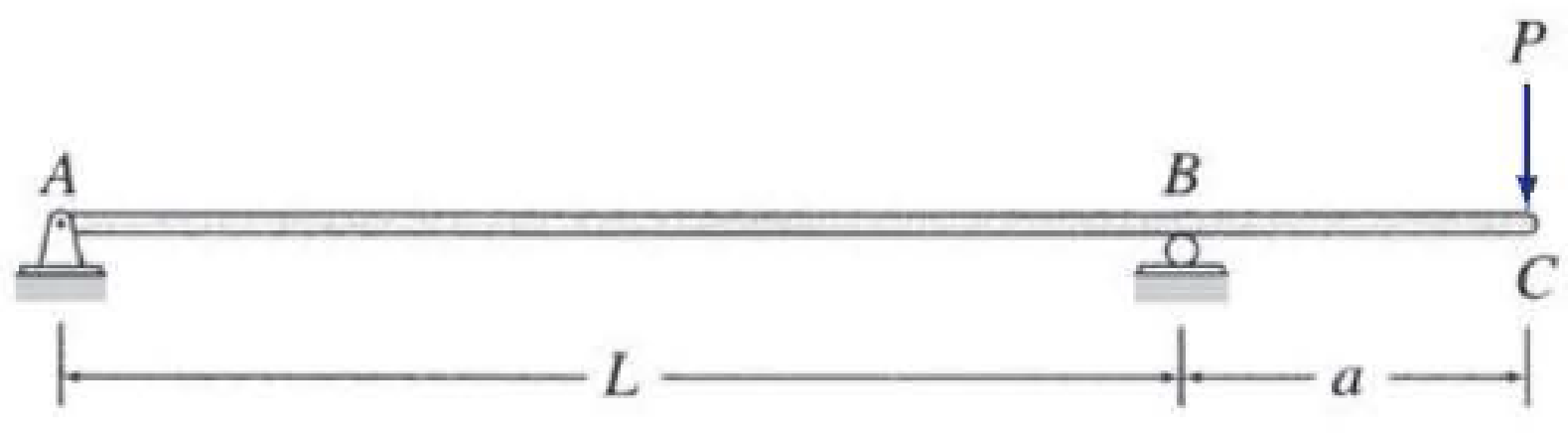# 6.1 through 6.6 Determine the equations for slope and deflection of the beam shown by the direct integration method. EI = constant. FIG. P6.6

#### Solutions

Chapter
Section
Chapter 6, Problem 6P
Textbook Problem
49 views

## 6.1 through 6.6 Determine the equations for slope and deflection of the beam shown by the direct integration method. EI = constant.FIG. P6.6To determine

Find the equations for slope and deflection of the beam using direct integration method.

### Explanation of Solution

Calculation:

Consider flexural rigidity EI of the beam is constant.

Draw the free body diagram of the beam as in Figure (1).

Refer Figure (1),

Consider upward force is positive and downward force is negative.

Consider clockwise  is negative and counterclowise is positive.

Determine the support reaction at A using the relation;

MB=0RA×L(P×a)=0RA=PaLRA=PaL()

Determine the support reaction at B using the relation;

V=0RA+RBP=0RB=P(PaL)RB=PL+PaLRB=P(1+aL)

Show the reaction values as in Figure (2).

Take a section at a distance of x.

Show the section as in Figure (2).

Consider the segment AB:

Refer Figure (2),

Write the equation for bending moment at x distance.

Mx=RA(x)=PaL(x)=PaxL

Write the equation for MEI as follows:

d2ydx2=1EI(PaxL)        (1)

Write the equation for slope as follows:

Integrate Equation (1) with respect to x.

dydx=θ=1EI(PaL)(xdx)=PaEIL(x22)+C1        (2)

Write the equation for deflection as follows:

Integrate Equation (2) with respect to x.

y=[PaEIL((x22))+C1]dx=Pa2EIL(x33)+C1x+C2        (3)

Find the integration constants C1andC2:

Write the boundary conditions as follows:

Atx=0;y=0:

Apply the above boundary conditions in Equation (3):

0=Pa2EIL((03)3)+C1(0)+C2C2=0

Write the boundary conditions as follows:

Atx=L;y=0:

Apply the above boundary conditions in Equation (3):

0=Pa2EIL(L33)+C1(L)+0C1(L)=PaL26EIC1=PaL6EI

Find the equation for slope.

Substitute PaL6EI for C1 in Equation (2).

θ=PaEIL(x22)+PaL6EI=PaL6EI(13x2L2)

Thus, the equation for slope is PaL6EI(13x2L2)_.

Find the equation for deflection.

Substitute PaL6EI for C1 and 0 for C2 in Equation (3).

y=Pa2EIL(x33)+(PaL6EI)x+0=Pa2EIL(x33)+(PaxL6EI)=PaxL6EI(1x2L2)

Thus, the equation for deflection is PaxL6EI(1x2L2)_.

Consider segment BC;

Show the distance at a distance of x as in Figure (4).

Refer Figure (2),

For segment BC the limit should be Lx(L+a).

Write the equation for bending moment at x distance.

Mx=P(L+ax)

Write the equation for MEI as follows:

d2ydx2=1EI(P(L+ax))=PEI(L+ax)        (4)

Write the equation for slope as follows:

Integrate Equation (4) with respect to x.

dydx=θ=PEI(L+ax)dx=PEI(Lx+axx22)+C3        (5)

Write the equation for deflection as follows:

Integrate Equation (5) with respect to x.

y=[PEI(Lx+axx22)+C3]dx=PEI(Lx22+ax22x36)+C3x+C4        (6)

Write the boundary conditions as follows:

Atx=L

The slope at left and right of support B is equal

### Still sussing out bartleby?

Check out a sample textbook solution.

See a sample solution

#### The Solution to Your Study Problems

Bartleby provides explanations to thousands of textbook problems written by our experts, many with advanced degrees!

Get Started

Find more solutions based on key concepts
For Problems 16.33 through 16.38, draw the isometric view of the following objects. Make the necessary measurem...

Engineering Fundamentals: An Introduction to Engineering (MindTap Course List)

Explain the difference between scaling up and scaling out.

Database Systems: Design, Implementation, & Management

Find the forces Q1,Q2, and Q3 so that the two forces systems are equivalent.

International Edition---engineering Mechanics: Statics, 4th Edition

Explain the need for the two-phase commit protocol. Then describe the two phases.

Database Systems: Design, Implementation, & Management

Explain the impact of the Internet on plagiarism.

Enhanced Discovering Computers 2017 (Shelly Cashman Series) (MindTap Course List)

If your motherboard supports ECC DDR3 memory, can you substitute non-ECC DDR3 memory?

A+ Guide to Hardware (Standalone Book) (MindTap Course List)

Name three methods used to perform welding, cutting, or brazing operations.

Welding: Principles and Applications (MindTap Course List)